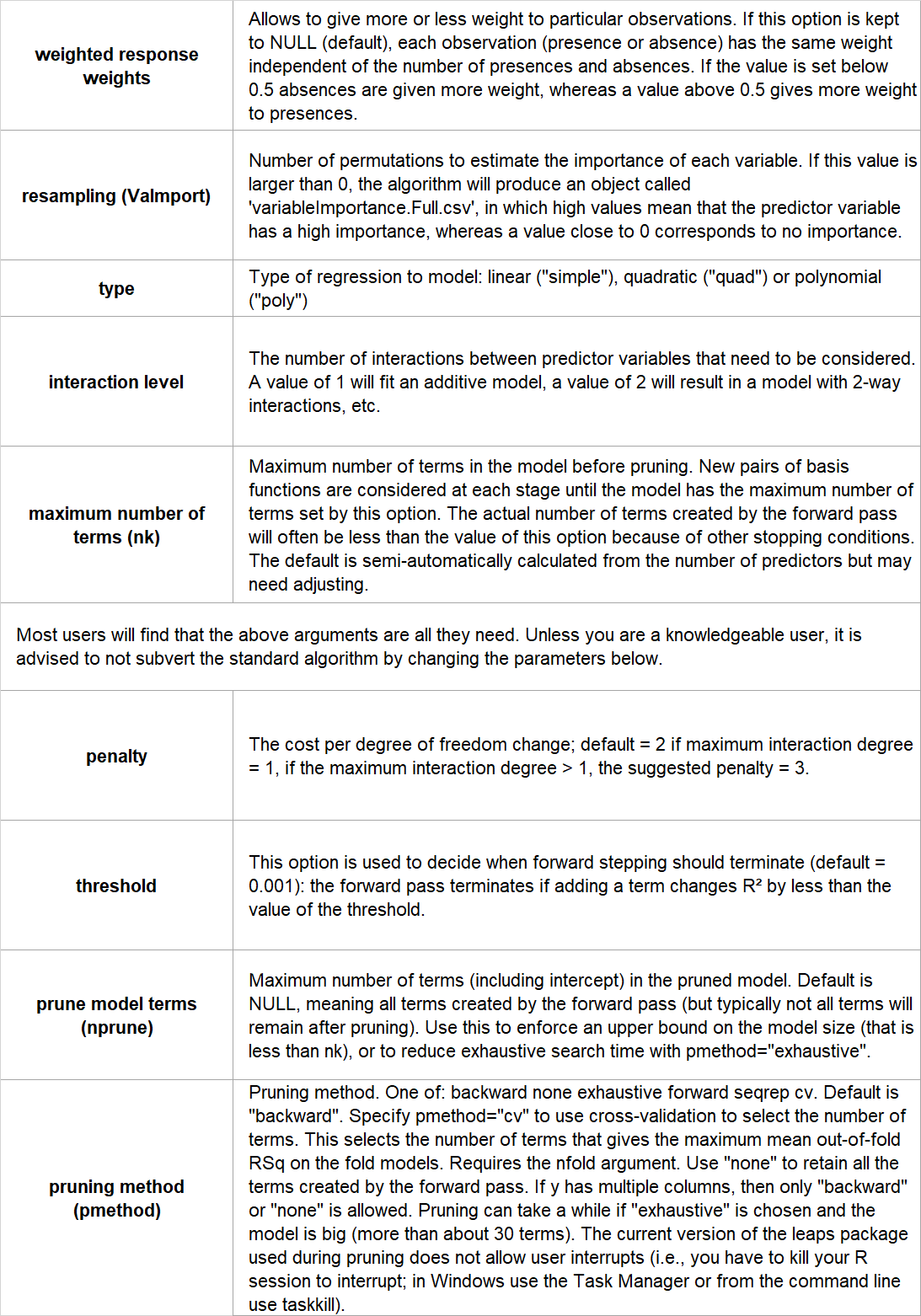### Introduction

Multivariate Adaptive Regression Splines (MARS) is a non-parametric regression method that builds multiple linear regression models across the range of predictor values. It does this by partitioning the data, and run a linear regression model on each different partition.

The MARS algorithm is an extension of linear models that makes no assumptions about the relationship between the response variable and the predictor variables. While Generalized Linear Models and Generalized Additive Models assume that the coefficients of the predictor variables are constant across all values of a predictor, the MARS algorithm specifically takes into account that this is often not the case. But the MARS algorithm also has similarities to machine learning models such as tree-based models, because it uses a similar iterative approach.

The MARS algorithm builds a model in two steps. First, it creates a collection of so-called basis functions (BF). In this procedure, the range of predictor values is partitioned in several groups. For each group, a separate linear regression is modeled, each with its own slope. The connections between the separate regression lines are called knots. The MARS algorithm automatically searches for the best spots to place the knots. Each knot has a pair of basis functions. These basis functions describe the relationship between the environmental variable and the response. The first basis function is ‘max(0, env var - knot), which means that it takes the maximum value out of two options: 0 or the result of the equation ‘environmental variable value – value of the knot’. The second basis function has the opposite form: max(0, knot - env var).For example, if the value of the environmental variable at the knot is 11, then:

Basis function 1: for any value below 11, the outcome of ‘Env var – Knot’ will result in a negative number, which is smaller than 0 and thus the outcome of the basis function is 0. This means that the outcome of basis function 1 is 0 for all environmental values up to the knot, while for all values after the knot, the outcome of basis function 1 is the value of the environmental variable minus 11.

Basis function 2: this has the opposite form, with the outcome of 0 for all environmental values after the knot, and the outcome of 11 minus value of environmental variable before the knot.

In the second step, MARS estimates a least-squares model with its basis functions as independent variables. It fits a very large model, that is subsequently pruned (like tree-based models) to avoid overfitting by iteratively removing basis functions that contribute the least to model fit.

• Works well with a large number of predictor variables
• Automatically detects interactions between variables
• It is an efficient and fast algorithm, despite its complexity
• Robust to outliers

### Limitations

• Susceptible to overfitting
• More difficult to understand and interpret than other methods
• Not good with missing data

### Assumptions

No assumptions are made about the distributions of the environmental variables. However, they should not be highly correlated with one another because this could cause problems with the estimation.

Yes.

### Configuration options

BCCVL uses the ‘earth’ package, implemented in biomod2. The user can set the following configuration options:### References

• Elith J, Graham CH, Anderson RP et al. (2006) Novel methods improve prediction of species’ distributions from occurrence data. Ecography, 29(2), 129-151.
• Franklin J (2010) Mapping species distributions: spatial inference and prediction. Cambridge University Press.
• Friedman JH (1991) Multivariate adaptive regression splines. The annals of statistics: 1-67.
• Hastie T, Tibshirani R, Friedman J (2009) The elements of statistical learning: data mining, inference and prediction. 2nd edition, Springer.
• Leathwick JR, Elith J, Hastie T (2006) Comparative performance of generalized additive models and multivariate adaptive regression splines for statistical modelling of species distributions. Ecological modelling, 199(2): 188-196.
• Milborrow S (2015) Notes on the earth package. http://www.milbo.org/doc/earth-notes.pdf